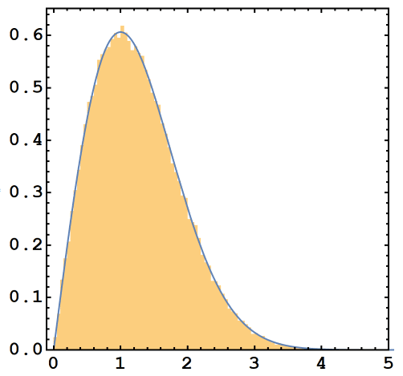# #StackBounty: #distributions #residuals #rayleigh Proof that \$f(t) P(x; sigma = 1)\$ is equivilant to \$P(x; sigma = f(t))\$ where \$P(x;…

### Bounty: 100

Consider some function or lineshape, $$f(t)$$, whose form is known. Also consider some one-parameter PDF, $$P(x;sigma)$$ where $$sigma$$ is a shape parameter — and importantly defines the mode of $$P(x;sigma)$$.

Now consider the following case. I now measure some data as a function of $$t$$ and I know that each measured point is sampled from the PDF $$P(x;sigma)$$, except now the shape parameter, $$sigma$$ is defined by $$f(t)$$. Such that
$$P(x;sigma = f(t))$$
That is, for every value of $$t$$ I measure my data, I am measuring a data point which is drawn from the PDF $$P(x;sigma)$$, but with a different shape parameter each time as $$sigma = f(t)$$.So I have some measured data
$$F(t)sim P(x;sigma = f(t))$$

If I now take the log of both my measured data and function, and subtract them
$$R_{rm{log}} = log_{10}(F) – log_{10}(f(t))$$
i.e. looking at the residuals, and converting back into a linear scale,
$$R_{rm{lin}} = 10^{R_{rm{log}}}$$, I find that the distribution of my residuals follow the distribution
$$P(x;sigma = 1)$$

How can I prove this mathematically?

I’ll show my scenario with some simulation. It is written in Mathematica but hopefully the code is

``````f[A_, w0_, t_] := A * Sin[w0 * t] + 2 * A
``````

I then generate my un-noised pure "fit function". I chose `A = 1`, `w0 = 0.1`

``````UnnoisedData =  Table[Log10[f[1, 0.1, t]], {t, 0 , 10, 10^-3}];
``````

This generates the noised data from `t = 0` to `t = 10` in a step size of `10^-3`. I now generate the noised data as

``````NoisedData = Table[Log10[RandomVariate[RayleighDistribution[f[1, 0.1, t]]]], {t, 0 , 100, 10^-3}];
``````

Here I have chosen the Rayleigh distribution and in Mathematica, the function `RayleighDistribution[s]` takes one argument `s` which is the shape parameter I described above. Notice in both cases I have already taken the logarithm to the base 10.

I know generate my residuals

``````Rlog = NoisedData - UnnoisedData;
Rlin = 10^Rlog;
``````

If I look at the histogram of my residuals $$Rlin$$ I seeSo my residual in linear units follow a perfect Rayeligh distribution with a shaoe parameter $$sigma = 1$$. I have confirmed this with large numbers of simulations. This example has $$100,001$$ data points, and perfomred Maximum Likelihood evaluations to confirm the shape parameter.

$$f(t) P(x;sigma = 1)$$
$$P(x; sigma = f(t))$$
because if we consider $$sigma$$ to be the mode of the PDF $$P(x; sigma)$$, then the two expressions above must be somehow equivalent.